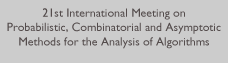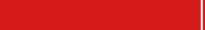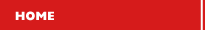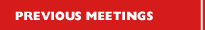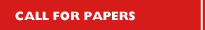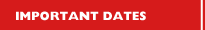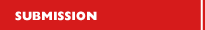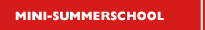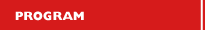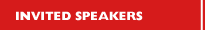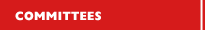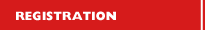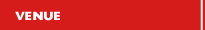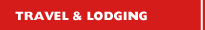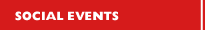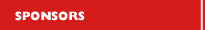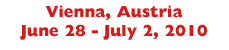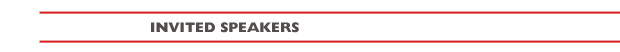The following speakters confirmed to give an invited talk: Noga Alon (Tel Aviv University): Color Coding: Variations and Applications Abstract: Color Coding is an algorithmic technique for  deciding efficiently if a given input graph contains a path of a given length (or another small subgraph of a certain type). It illustrates  well the phenomenon that probabilistic reasoning can be helpful in the design of deterministic algorithms.  Applications of the method in computational biology motivate the study of similar algorithms for counting the number of copies of a given subgraph. While it is unlikely that exact counting of this type can be performed efficiently, approximate counting is possible, and leads to the investigation of an intriguing variant of families of perfect hash functions. I will describe the motivation and the new results on this topic, including a recent variant called chromatic coding. The new results are based on joint papers with Gutner and with Lokshtanov and Saurabh. Yuliy Baryshnikov (Bell Laboratories): Search on the brink of chaos. Abstract: Search on the half-line is one of the simplest instances of the search problems, going back to Bellman '63 and Beck '64. Deceptive simplicity of the average case of this problem hides  behind it an intricate structure - namely that of a Hamiltonian mapping with coexisting chaotic and regular dynamics, unbounded invariant domains and other unexpected pathologies. (Joint work with Vadim Zharnitsky, UIUC) Daniel Panario (Carleton University): Polynomials over Finite Fields: Algorithms and Randomness Abstract: The central objects of this talk are univariate polynomials over finite fields. We review a methodology from analytic combinatorics that allows the study of algorithmic properties, as well as the derivation of average-case analysis for these algorithms. This methodology can be naturally used to provide precise information on the decomposition of polynomials into its irreducible factors, similar to the classical problem of the decomposition of integers into primes. Examples of these results are provided. The shape of a random univariate polynomial over a finite field is also given. Then, we briefly show several results for random polynomials over finite fields that were not obtained using the above methodology from analytic combinatorics. We finish with some open problems from actual research areas in finite fields where univariate polynomials play a central role but no technique from analytic combinatorics have been successfully employed yet. Oliver Riordan (University of Oxford): Random graphs are the natural mathematical models for a wide variety of networks in the real world. However, in many cases the classical random graph models introduced 50 years ago are not appropriate: one reason is that real networks tend to be highly inhomogeneous, with wide variations between the degrees of different nodes, for example. This has led to the introduction of many new inhomogeneous models over the last decade, with perhaps the best known being the Barabási--Albert growth with preferential attachment model. Many of these models have been studied individually, but they are too varied to study together. However, a few years ago Bollobás, Janson and Riordan introduced a very general (class of) inhomogeneous model(s) that is general enough to include or approximate many of these earlier models, but simple enough for mathematical analysis. The talk with discuss this and related models, focussing on results related to percolation, i.e., involving large-scale behaviour. There are intriguing connections between these models and the work of Borgs, Chayes, Lov\'asz, S\'os, Szegedy and Vesztergombi on metrics for {\sl dense} graphs, which will also be discussed briefly. Peter Winkler (Dartmouth): The Automaton as Statistician Abstract: How reliably can an automaton determine whether a coin is heads-biased or tails-biased?  This calculation, which arises in the study of pseudo-random number generation as well as in automata theory, turns out to be more subtle than expected.  Joint work with Dartmouth graduate student Joshua Brody.